## Friday, November 18, 2016

### Updates to permit user control of line end types in `plotSimmap`

I recently made a couple of small updates to `plotSimmap` - firstly, to allow user control of the line end type (`lend`, as in `par`); and secondly, for `type="fan"`, to plot the two edges originating from the root as a single segmented line.

Here is a quick example using the latest version of phytools on GitHub:

``````library(phytools)
packageVersion("phytools")
``````
``````##  '0.5.59'
``````
``````data(anoletree)
plot(anoletree,lwd=5,ftype="i",fsize=0.5)
``````
``````## no colors provided. using the following legend:
##        CG        GB        TC        TG        Tr        Tw
##   "black"     "red"  "green3"    "blue"    "cyan" "magenta"
``````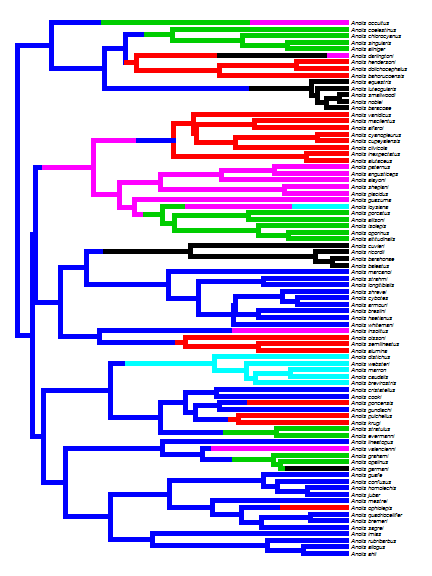``````plot(anoletree,lwd=5,ftype="i",fsize=0.5,lend=0) ## curved
``````
``````## no colors provided. using the following legend:
##        CG        GB        TC        TG        Tr        Tw
##   "black"     "red"  "green3"    "blue"    "cyan" "magenta"
``````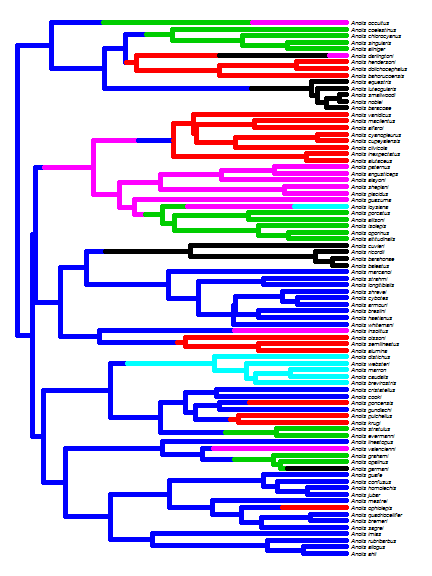``````plot(anoletree,lwd=5,ftype="i",fsize=0.5,lend=1) ## mitered
``````
``````## no colors provided. using the following legend:
##        CG        GB        TC        TG        Tr        Tw
##   "black"     "red"  "green3"    "blue"    "cyan" "magenta"
``````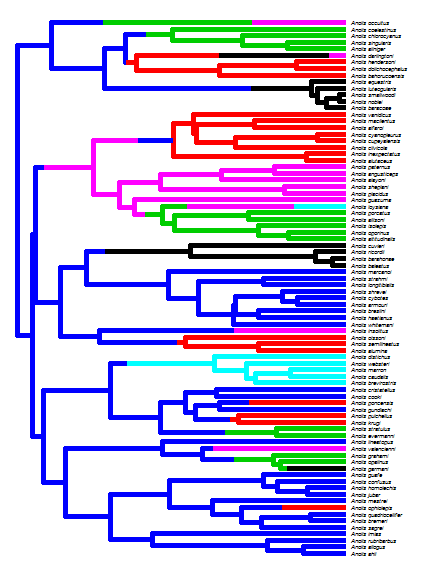Or as `type="fan"`

``````plot(anoletree,lwd=5,ftype="i",fsize=0.8,lend=1,type="fan")
``````
``````## no colors provided. using the following legend:
##        CG        GB        TC        TG        Tr        Tw
##   "black"     "red"  "green3"    "blue"    "cyan" "magenta"
``````
``````cols<-setNames(palette()[1:length(unique(getStates(anoletree,
"tips")))],
sort(unique(getStates(anoletree,"tips"))))
y=0.9*par()\$usr,prompt=FALSE,fsize=0.9)
``````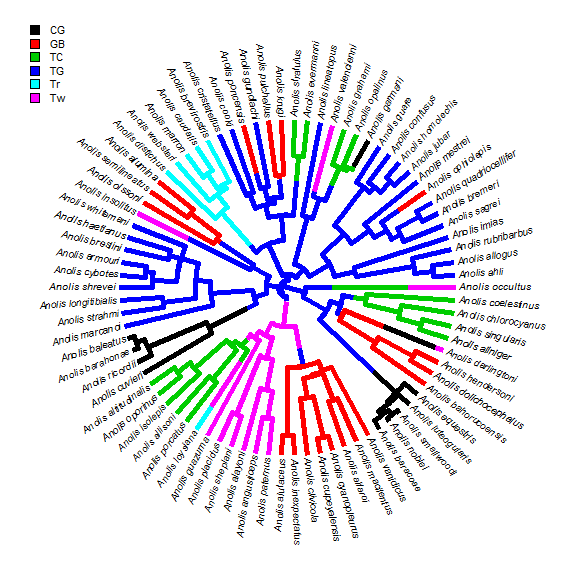Note that the 'pixelation' (aka. aliasing) is just an effect of the rendering and disappears if the plot is rendered as a PDF, e.g.: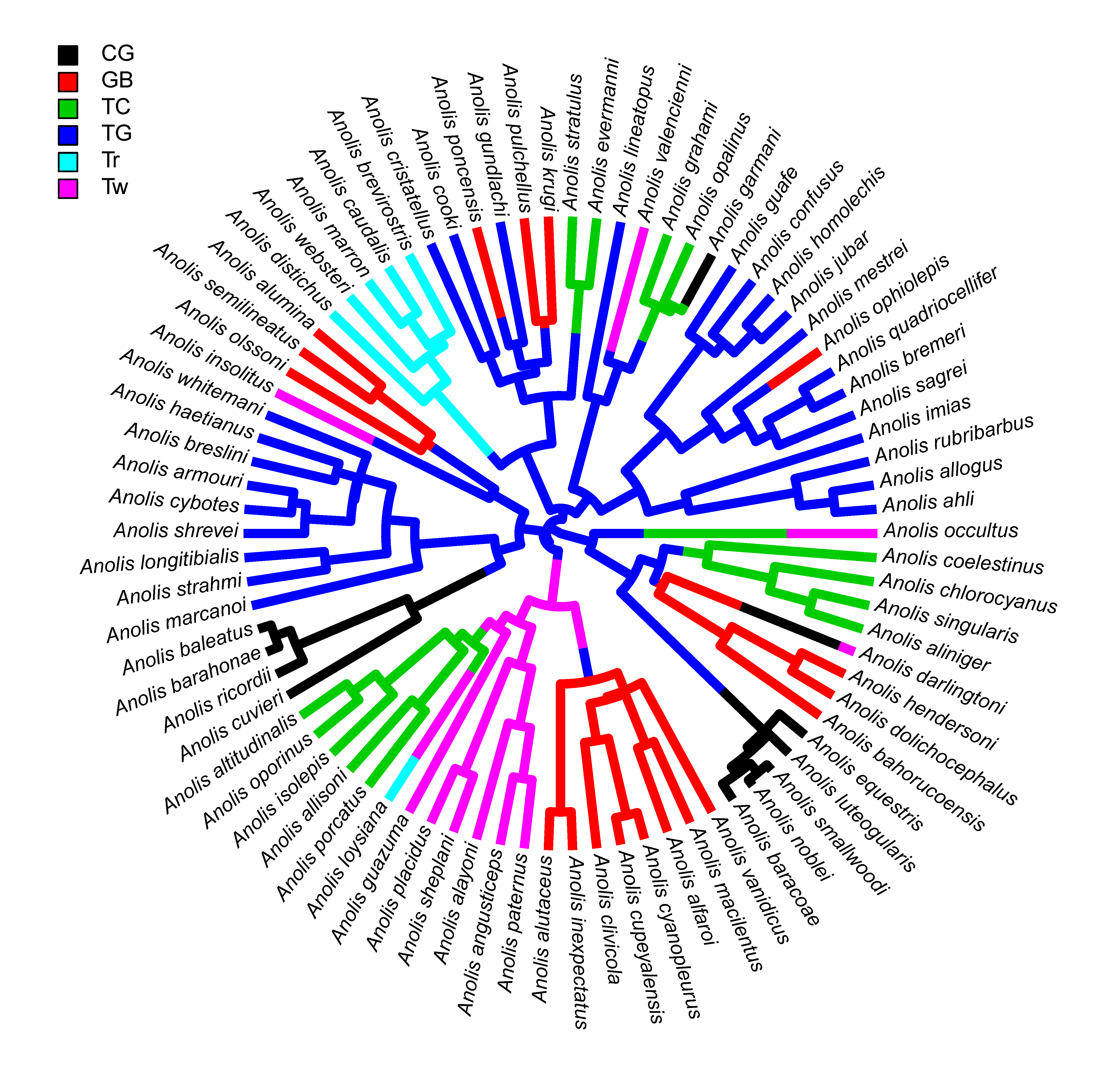Finally, the purpose of plotting the two lines originating at the root as a single segmented line is the eliminate what can be an ugly overlap of the two daughter edges when plotted separately:

``````plotTree(tree,type="fan",lwd=4,part=0.5,lend=1,fsize=0.5)
``````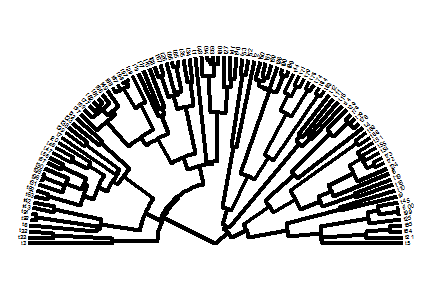or: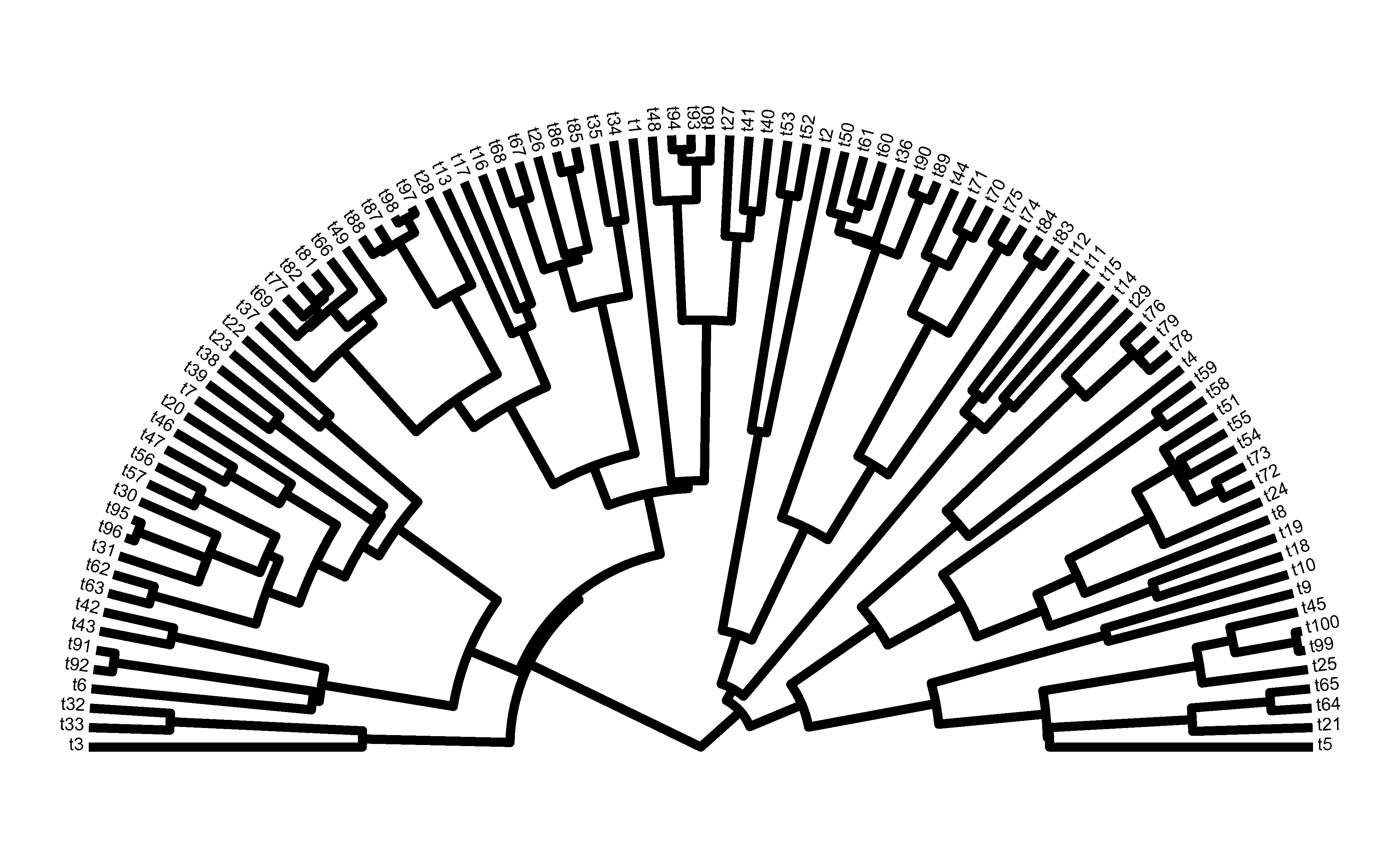(rendered more nicely).

That's it.

1.Hi, Liam,

I was wondering if it is possible to input an ultrametric tree to a make.simmap function for stochastic mapping.

Thank you,
Andrea

1.Sure, of course. In fact, I would recommend that.

Note: due to the very large amount of spam, all comments are now automatically submitted for moderation.